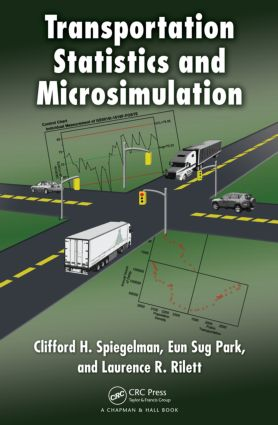Transportation Statistics and Microsimulation

1st Edition

Chapman and Hall/CRC

384 pages | 80 B/W Illus.

Purchasing Options:\$ = USD
Hardback: 9781439800232
pub: 2010-10-18
SAVE ~\$14.59
\$72.95
\$58.36
x
eBook (VitalSource) : 9780429108952
pub: 2016-04-19
from \$36.48

FREE Standard Shipping!

Description

By discussing statistical concepts in the context of transportation planning and operations, Transportation Statistics and Microsimulation provides the necessary background for making informed transportation-related decisions. It explains the why behind standard methods and uses real-world transportation examples and problems to illustrate key concepts.

The Tools and Methods to Solve Transportation Problems

Classroom-tested at Texas A&M University, the text covers the statistical techniques most frequently employed by transportation and pavement professionals. To familiarize readers with the underlying theory and equations, it contains problems that can be solved using statistical software. The authors encourage the use of SAS’s JMP package, which enables users to interactively explore and visualize data. Students can buy their own copy of JMP at a reduced price via a postcard in the book.

Practical Examples Show How the Methods Are Used in Action

Drawing on the authors’ extensive application of statistical techniques in transportation research and teaching, this textbook explicitly defines the underlying assumptions of the techniques and shows how they are used in practice. It presents terms from both a statistical and a transportation perspective, making conversations between transportation professionals and statisticians smoother and more productive.

Reviews

In this treatment of statistics specifically directed to transportation planners and engineers, Spiegelman and other transportation experts discuss the basics of statistical and graphical methods, differences between methodologies, strategies for conducting computer-aided statistical designs (using JMP software by SAS), bias-corrected confidence intervals, re-sampling techniques for evaluating uncertainties, and the concepts of Bayesian estimation and smoothing estimators. They also overview increasingly used traffic microsimulation models. The text includes homework problems, appended information on soft modeling and nonparametric model building, and a companion website for access to data sets.

SciTech Book News, February 2011

Overview: The Role of Statistics in Transportation Engineering

What Is Engineering?

What Is Transportation Engineering?

Goal of the Textbook

Overview of the Textbook

Who Is the Audience for This Textbook?

Relax—Everything Is Fine

Graphical Methods for Displaying Data

Introduction

Histogram

Box and Whisker Plot

Quantile Plot

Scatter Plot

Parallel Plot

Time Series Plot

Quality Control Plots

Concluding Remarks

Numerical Summary Measures

Introduction

Measures of Central Tendency

Measures of Relative Standing

Measures of Variability

Measures of Association

Concluding Remarks

Probability and Random Variables

Introduction

Sample Spaces and Events

Interpretation of Probability

Random Variable

Expectations of Random Variables

Covariances and Correlation of Random Variables

Computing Expected Values of Functions of Random Variables

Conditional Probability

Bayes’ Theorem

Concluding Remarks

Common Probability Distributions

Introduction

Discrete Distributions

Continuous Distributions

Concluding Remarks

Appendix: Table of the Most Popular Distributions in Transportation Engineering

Sampling Distributions

Introduction

Random Sampling

Sampling Distribution of a Sample Mean

Sampling Distribution of a Sample Variance

Sampling Distribution of a Sample Proportion

Concluding Remarks

Inferences: Hypothesis Testing and Interval Estimation

Introduction

Fundamentals of Hypothesis Testing

Inferences on a Single Population Mean

Inferences about Two Population Means

Inferences about One Population Variance

Inferences about Two Population Variances

Concluding Remarks

Appendix: Welch (1938) Degrees of Freedom for the Unequal Variance t-Test

Other Inferential Procedures: ANOVA and Distribution-Free Tests

Introduction

Comparisons of More than Two

Population Means

Multiple Comparisons

One- and Multiway ANOVA

Assumptions for ANOVA

Distribution-Free Tests

Conclusions

Inferences Concerning Categorical Data

Introduction

Tests and Confidence Intervals for a Single Proportion

Tests and Confidence Intervals for Two Proportions

Chi-Square Tests Concerning More Than Two Population Proportions

The Chi-Square Goodness-of-Fit Test for Checking Distributional Assumptions

Conclusions

Linear Regression

Introduction

Simple Linear Regression

Transformations

Understanding and Calculating R2

Verifying the Main Assumptions in Linear Regression

Comparing Two Regression Lines at a Point and Comparing Two Regression Parameters

The Regression Discontinuity Design (RDD)

Multiple Linear Regression

Variable Selection for Regression Models

Concluding Remarks

Regression Models for Count Data

Introduction

Poisson Regression Model

Overdispersion

Assessing Goodness of Fit of Poisson Regression Models

Negative Binomial Regression Model

Concluding Remarks

Appendix: Maximum Likelihood Estimation

Experimental Design

Introduction

Comparison of Direct Observation and Designed Experiments

Motivation for Experimentation

A Three-Factor, Two Levels per Factor Experiment

Factorial Experiments

Fractional Factorial Experiments

Screening Designs

D-Optimal and I-Optimal Designs

Sample Size Determination

Field and Quasi-Experiments

Concluding Remarks

Appendix: Choice Modeling of Experiments

Cross-Validation, Jackknife, and Bootstrap Methods for Obtaining Standard Errors

Introduction

Methods for Standard Error Estimation When a Closed-Form Formula Is Not Available

Cross-Validation

The Jackknife Method for Obtaining Standard Errors

Bootstrapping

Concluding Remarks

Bayesian Approaches to Transportation Data Analysis

Introduction

Fundamentals of Bayesian Statistics

Bayesian Inference

Concluding Remarks

Microsimulation

Introduction

Overview of Traffic Microsimulation Models

Analyzing Microsimulation Output

Performance Measures

Concluding Remarks

Appendix: Soft Modeling and Nonparametric Model Building

Homework Problems and References appear at the end of each chapter.

Clifford Spiegelman is a distinguished professor of statistics at Texas A&M University, where he has been for twenty-three years. Dr. Spiegelman is also a senior research scientist at the Texas Transportation Institute.

Eun Sug Park is a research scientist at the Texas Transportation Institute. Dr. Park was a recipient of the TRB Pedestrian Committee Outstanding Paper Award (2006 and 2009) and the Patricia Waller Award (2009).

Laurence R. Rilett is a distinguished professor of civil engineering at the University of Nebraska–Lincoln. He also is the director of both the U.S. Department of Transportation’s Region VII University Transportation Center and the Nebraska Transportation Center.